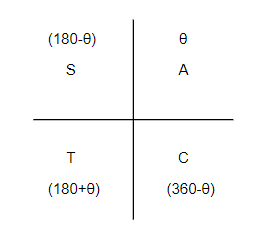QuestionAnswers

# Find the general solution of $\csc x=-2$.Verified
112.5K+ Views
Hint: First of all, we can write $\csc x=\dfrac{1}{\sin x}$. Now, we can find the principal solution of $\sin x=\dfrac{-1}{2}$. By using the principal solution, we can find the general solution using $\sin x=\sin \alpha$, then $x=n\pi +{{\left( -1 \right)}^{n}}\alpha$.

Here we have to find the general solution of $\csc x=-2$. Let us consider the equation given in the question, $\csc x=-2$. We know that $\csc x=\dfrac{1}{\sin x}$, so we get, $\dfrac{1}{\sin x}=-2$. By cross multiplying the equation, we get, $\sin x=\dfrac{-1}{2}$. We know that $\sin 30{}^\circ =\dfrac{1}{2}$. Since $x$ is negative, it will be in the third and fourth quadrants.Value in the third quadrant $=180{}^\circ +30{}^\circ =210{}^\circ$. Value in the fourth quadrant $=360{}^\circ -30{}^\circ =330{}^\circ$. So, for $\sin x=\dfrac{-1}{2}$, we get,
\begin{align} & x=210{}^\circ =210\times \dfrac{\pi }{180}=\dfrac{7\pi }{6} \\ & x=330{}^\circ =330\times \dfrac{\pi }{180}=\dfrac{11\pi }{6} \\ \end{align}
Now, to find the general solution, let $\sin x=\sin \theta \ldots \ldots \ldots \left( i \right)$
And we know that $\sin x=\dfrac{-1}{2}\ldots \ldots \ldots \left( ii \right)$
From equation (i) and equation (ii), we get,
$\sin \theta =\dfrac{-1}{2}\ldots \ldots \ldots \left( iii \right)$
We have already calculated, $\sin \dfrac{7\pi }{6}=\dfrac{-1}{2}\ldots \ldots \ldots \left( iv \right)$
So, from the equations, (i), (iii) and (iv), we get,
\begin{align} & \sin x=\sin \theta =\sin \dfrac{7\pi }{6} \\ & \Rightarrow \sin x=\sin \dfrac{7\pi }{6} \\ \end{align}
We know that when $\sin x=\sin \theta$, then $x=n\pi +{{\left( -1 \right)}^{n}}\theta$. By using this relation, we get, $x=n\pi +{{\left( -1 \right)}^{n}}\dfrac{7\pi }{6}$, when $n\in N$.
Hence, we get the general solution of $\csc x=-2$ as $x=n\pi +{{\left( -1 \right)}^{n}}\dfrac{7\pi }{6}$.

Note: In these types of questions, instead of remembering all the multiple formulas for each trigonometric ratio, it is better to convert sec, cosec and cot into cos, sin and tan respectively and then solve the question. It is also advised to learn the values of sin, cos, and tan at the standard angles which are, $0{}^\circ ,30{}^\circ ,45{}^\circ ,60{}^\circ ,90{}^\circ$ as we can always find the higher angles with the use of these angles.